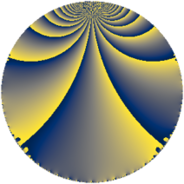# Properties

 Label 441.4.wLevel $441$ Weight $4$ Character orbit 441.w Rep. character $\chi_{441}(62,\cdot)$ Character field $\Q(\zeta_{14})$ Dimension $336$ Sturm bound $224$

# Related objects

## Defining parameters

 Level: $$N$$ $$=$$ $$441 = 3^{2} \cdot 7^{2}$$ Weight: $$k$$ $$=$$ $$4$$ Character orbit: $$[\chi]$$ $$=$$ 441.w (of order $$14$$ and degree $$6$$) Character conductor: $$\operatorname{cond}(\chi)$$ $$=$$ $$147$$ Character field: $$\Q(\zeta_{14})$$ Sturm bound: $$224$$

## Dimensions

The following table gives the dimensions of various subspaces of $$M_{4}(441, [\chi])$$.

Total New Old
Modular forms 1032 336 696
Cusp forms 984 336 648
Eisenstein series 48 0 48

## Trace form

 $$336q + 224q^{4} + 44q^{7} + O(q^{10})$$ $$336q + 224q^{4} + 44q^{7} - 896q^{16} - 336q^{22} - 1904q^{25} - 728q^{28} - 1008q^{34} - 1204q^{37} - 1008q^{40} + 448q^{43} + 840q^{46} + 1720q^{49} + 112q^{52} - 1260q^{55} - 504q^{58} - 9548q^{61} + 9128q^{64} - 3472q^{67} - 1620q^{70} + 4284q^{76} + 2072q^{79} + 12180q^{82} + 4368q^{85} - 5040q^{88} + 5952q^{91} + 3192q^{94} + O(q^{100})$$

## Decomposition of $$S_{4}^{\mathrm{new}}(441, [\chi])$$ into newform subspaces

The newforms in this space have not yet been added to the LMFDB.

## Decomposition of $$S_{4}^{\mathrm{old}}(441, [\chi])$$ into lower level spaces

$$S_{4}^{\mathrm{old}}(441, [\chi]) \cong$$ $$S_{4}^{\mathrm{new}}(147, [\chi])$$$$^{\oplus 2}$$